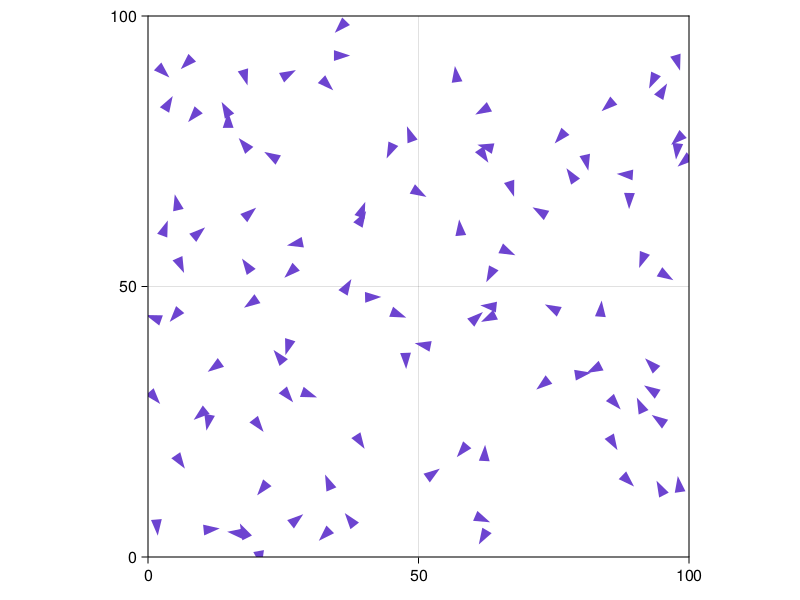# Flocking model

The flock model illustrates how flocking behavior can emerge when each bird follows three simple rules:

• maintain a minimum distance from other birds to avoid collision
• fly towards the average position of neighbors
• fly in the average direction of neighbors

It is also available from the Models module as Models.flocking.

## Defining the core structures

We begin by calling the required packages and defining an agent type representing a bird.

using Agents, LinearAlgebra

mutable struct Bird <: AbstractAgent
id::Int
pos::NTuple{2,Float64}
vel::NTuple{2,Float64}
speed::Float64
cohere_factor::Float64
separation::Float64
separate_factor::Float64
match_factor::Float64
visual_distance::Float64
end

The fields id and pos are required for every agent. The field vel is required for using move_agent! in ContinuousSpace. speed defines how far the bird travels in the direction defined by vel per step. seperation defines the minimum distance a bird must maintain from its neighbors. visual_distance refers to the distance a bird can see and defines a radius of neighboring birds. The contribution of each rule defined above recieves an importance weight: cohere_factor is the importance of maintaining the average position of neighbors, match_factor is the importance of matching the average trajectory of neighboring birds, and separate_factor is the importance of maining the minimum distance from neighboring birds.

The function initialize_model generates birds and returns a model object using default values.

function initialize_model(;
n_birds = 100,
speed = 1.0,
cohere_factor = 0.25,
separation = 4.0,
separate_factor = 0.25,
match_factor = 0.01,
visual_distance = 5.0,
extent = (100, 100),
)
space2d = ContinuousSpace(extent; spacing = visual_distance/1.5)
model = ABM(Bird, space2d, scheduler = Schedulers.Randomly())
for _ in 1:n_birds
vel = Tuple(rand(model.rng, 2) * 2 .- 1)
model,
vel,
speed,
cohere_factor,
separation,
separate_factor,
match_factor,
visual_distance,
)
end
return model
end

## Defining the agent_step!

agent_step! is the primary function called for each step and computes velocity according to the three rules defined above.

function agent_step!(bird, model)
# Obtain the ids of neighbors within the bird's visual distance
neighbor_ids = nearby_ids(bird, model, bird.visual_distance)
N = 0
match = separate = cohere = (0.0, 0.0)
# Calculate behaviour properties based on neighbors
for id in neighbor_ids
N += 1
neighbor = model[id].pos

# cohere computes the average position of neighboring birds
if euclidean_distance(bird.pos, neighbor, model) < bird.separation
# separate repels the bird away from neighboring birds
end
# match computes the average trajectory of neighboring birds
match = match .+ model[id].vel
end
N = max(N, 1)
# Normalise results based on model input and neighbor count
cohere = cohere ./ N .* bird.cohere_factor
separate = separate ./ N .* bird.separate_factor
match = match ./ N .* bird.match_factor
# Compute velocity based on rules defined above
bird.vel = (bird.vel .+ cohere .+ separate .+ match) ./ 2
bird.vel = bird.vel ./ norm(bird.vel)
# Move bird according to new velocity and speed
move_agent!(bird, model, bird.speed)
end
agent_step! (generic function with 1 method)

## Plotting the flock

using InteractiveDynamics
using CairoMakie

The great thing about abmplot is its flexibility. We can incorporate the direction of the birds when plotting them, by making the "marker" function am create a Polygon: a triangle with same orientation as the bird's velocity. It is as simple as defining the following function:

const bird_polygon = Polygon(Point2f[(-0.5, -0.5), (1, 0), (-0.5, 0.5)])
function bird_marker(b::Bird)
φ = atan(b.vel, b.vel) #+ π/2 + π
scale(rotate2D(bird_polygon, φ), 2)
end
bird_marker (generic function with 1 method)

Where we have used the utility functions scale and rotate2D to act on a predefined polygon. We now give bird_marker to abmplot, and notice how the as keyword is meaningless when using polygons as markers.

model = initialize_model()
figure, = abmplot(model; am = bird_marker)
figureAnd let's also do a nice little video for it:

abmvideo(
"flocking.mp4", model, agent_step!;
am = bird_marker,
framerate = 20, frames = 100,
title = "Flocking"
)